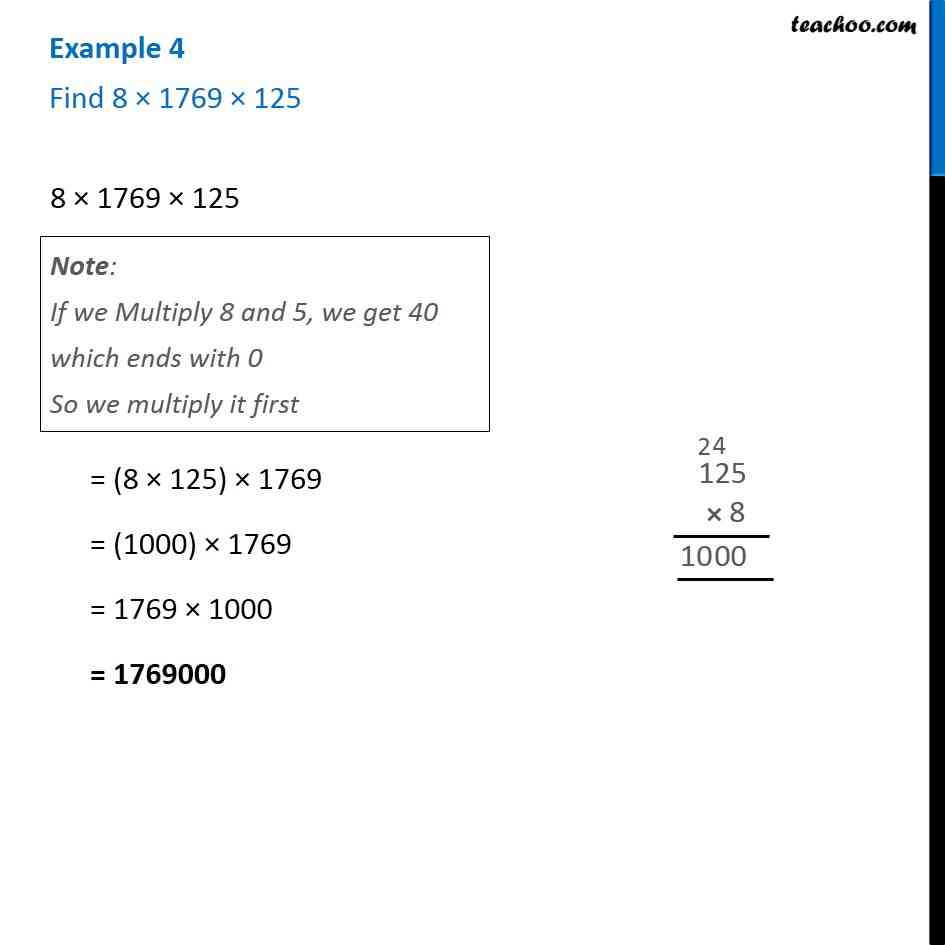Examples

Chapter 2 Class 6 Whole Numbers
Serial order wiseGet live Maths 1-on-1 Classs - Class 6 to 12

### Transcript

Example 4 - Chapter 2 Class 6 Whole Numbers - NCERT Book Find 8 × 1769 × 125 8 × 1769 × 125 Note: If we multiply 8 and 5, we get 40 which ends with 0 So, we multiply them first = (8 × 125) × 1769 = (1000) × 1769 = 1769 × 1000 = 1769000